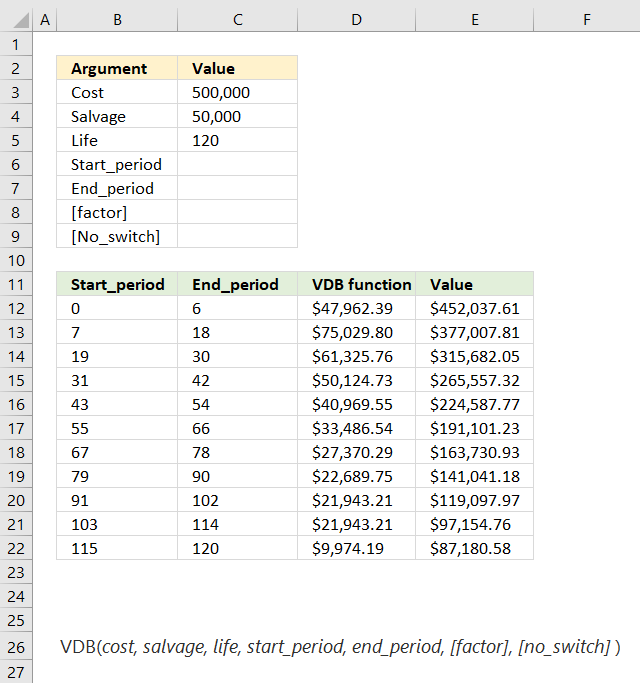Author: Oscar Cronquist Article last updated on May 11, 2022The VDB function calculates the depreciation of an asset for a given period using the double-declining balance method or based on user input, you may use partial periods in this function. VDB is an abbreviation for variable declining balance.

Formula in cell D12:

=VDB(C3,C4,C5,B12,C12)

### Excel Function Syntax

VDB(cost, salvage, life, start_period, end_period, [factor], [no_switch])

### Arguments

 Cost Required. What you pay for the asset. Salvage Required. The value of the asset at the end of depreciation. Life Required. The number of periods the asset is being depreciated. Start_period Required. The start of the range you want to calculate the depreciation. Start_period must use the same units as life. End_period Required. The end of the range you want to calculate the depreciation. End_period must use the same units as life. [factor] Optional. How quickly the balance declines, default value is 2 (the double-declining balance method). [no-switch] Optional.  A boolean value determining whether to use to straight-line depreciation when depreciation is larger than the declining balance calculation. TRUE - Does not switch to a straight-line depreciation even if the depreciation is larger than the declining balance calculation. FALSE - Switches to straight-line depreciation if the depreciation is larger than the declining balance calculation.